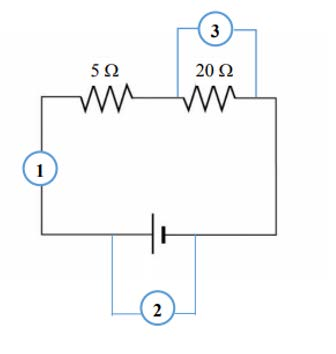# Solutions to the Quiz on Electric Circuits, Voltage, Ohm's Law, and Power

Question 1: A current of 60 mA flows through a wire for 1 minute,
Calculate the quantity of charge moved by the current during this time?
• 2.6 C
• 3.6 C
• 4.6 C
• 4.0 C
Explanation: Charge q = I × t and 1 C = 1 A•s, and hence q = 0.06A × 60s = 3.6 C

Question 2: An battery is used to ..................

• not to maintain a potential difference.
• maintain a potential difference.
• maintain a potential velocity.
• keep the mass the same.

Question 3: Assume a resistor R is connected across a battery of negligible internal resistance.
If the resistance of R is halved, the current in R would be..................

• halfed.
• doubled.
• zero.
• the same as before.

Explanation: We know that current I = ε / R, and so if R is halved then I would be doubled.

Question 4: Let's consider a 9-V battery in a simple circuit generating a current of 2A through the circuit.
Calculate the power being delivered by the battery.
• 0 watt
• 9 watts
• 8 watts
• 18 watts

Explanation:
Power P = ε × I and so P = 9 × 2 = 18 watts

Question 5: In the circuit shown below, which device is used to measure the electric current in the circuit?• 1
• 2
• 3
• none

Question 6: Assume that a student would like to measure the voltage across one of the resistors in the circuit shown above (question 5),
the student would connect a voltmeter ..........................the resistor.

• in parallel with
• in perpendicular with
• opposite to
• in any position with

Question 7: The resistance of a circuit element is a measure of ................

• the amount of work that an electron can perform.
• the ratio of the voltage difference between element ends to the current in the element.
• energy
• power

Question 8: Consider a circuit where the voltage drop across a resistor is 8.0 V for a current of 2.0 A in the resistor.
Calculate the current that will generate a voltage drop of 4.0 V across the resistor.

• 1 A
• 2 A
• 3 A
• 0 A
Explanation: We know that current I = ε / R, and so if ε is halved then I would be also halved and so equal to 1 A.

Question 9: Ohm's law tells us that the amount of current generated in a circuit is..........................

• directly proportional to resistance.
• directly proportional to voltage.
• directly proportional to current.
• inversely proportional to voltage.